# RD Sharma Solutions For Class 12 Maths Exercise 19.20 Chapter 19 Indefinite Integrals

RD Sharma Solutions for Class 12 Maths Exercise 19.20 Chapter 19 Indefinite Integrals is provided here. In this exercise, we shall discuss how to examine the integrals of the form

$$\begin{array}{l}\int \frac{P(x)}{ax^{2}+bx+c}\ dx\end{array}$$
where P(x) is a polynomial of degree two or more. For students desiring an after-school reference for solving the exercise problems, the RD Sharma Solutions for Class 12 Mathematics is the best resource a student can get.

All the solutions are created by subject experts at BYJU’S, keeping in mind the latest CBSE marking patterns. RD Sharma Solutions for Class 12 Maths Chapter 19 Exercise 19.20 PDF can be downloaded easily from the link below.

## Download the PDF of RD Sharma Solutions For Class 12 Chapter 19 – Indefinite Integrals Exercise 19.20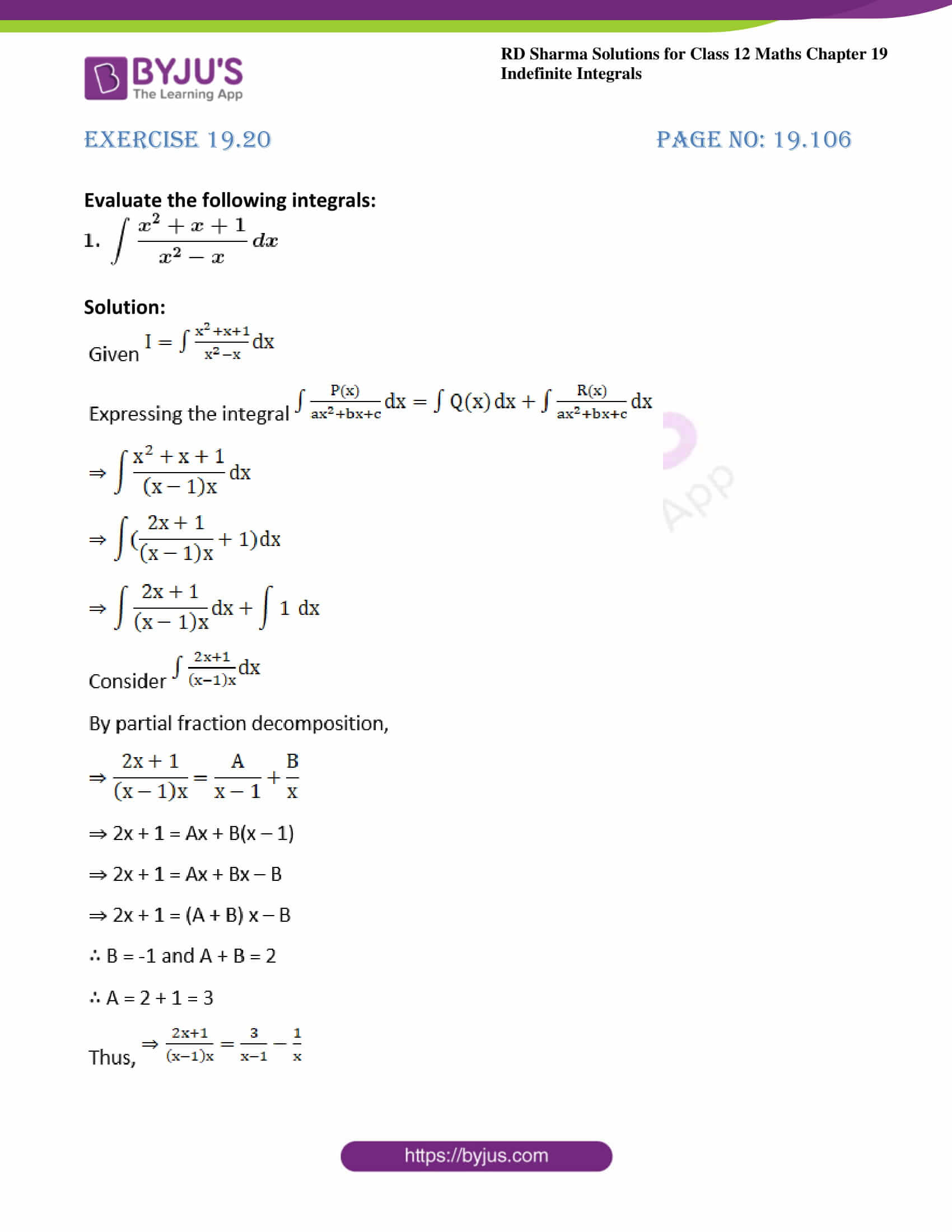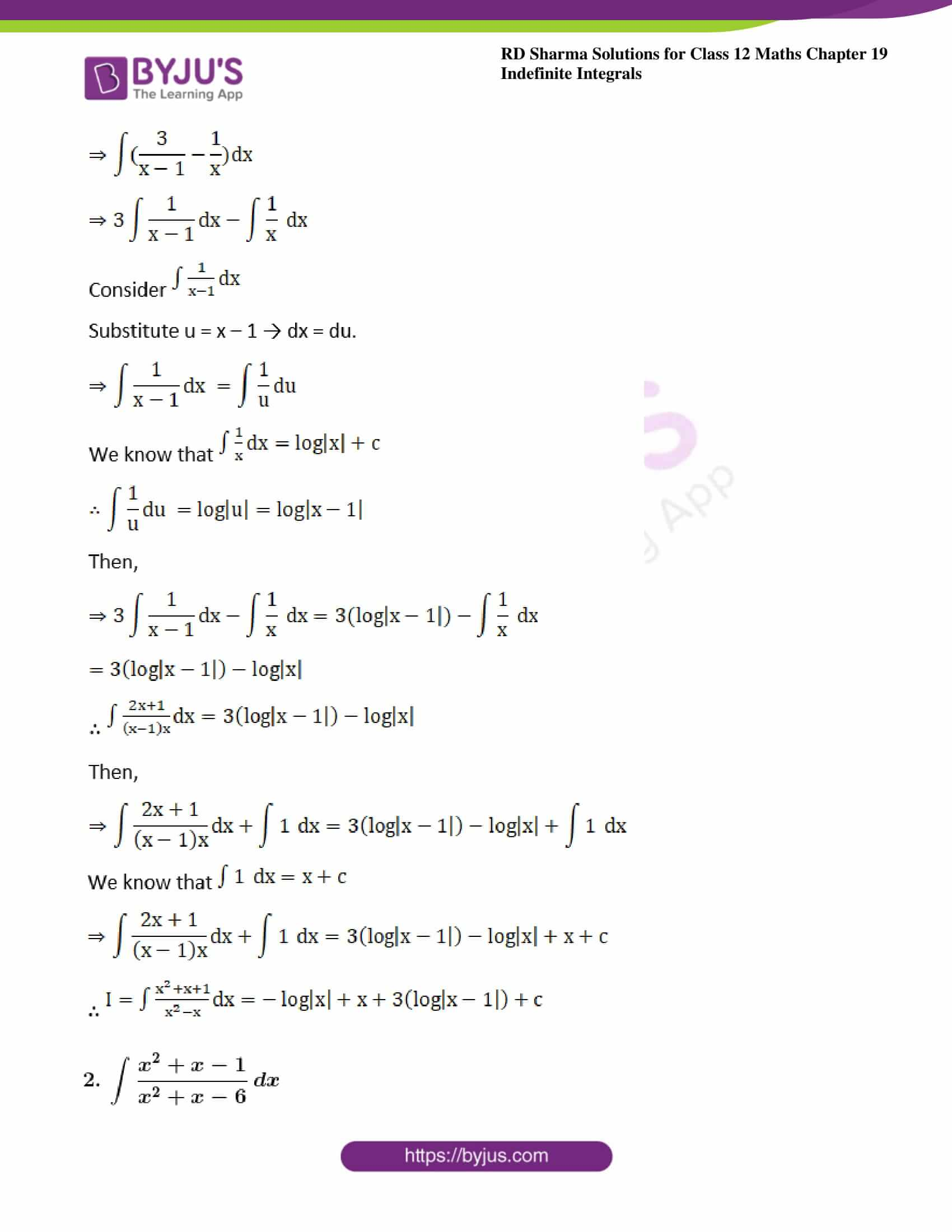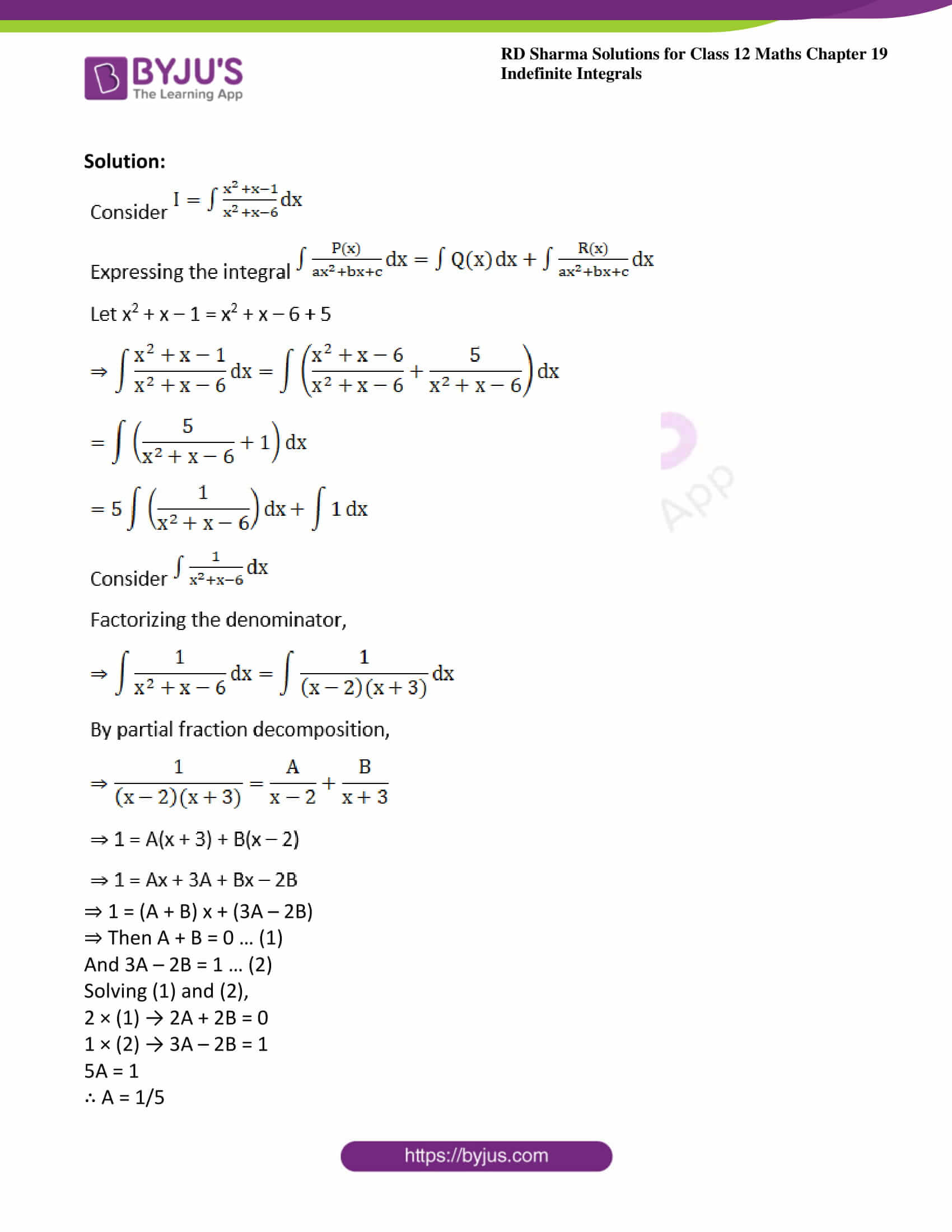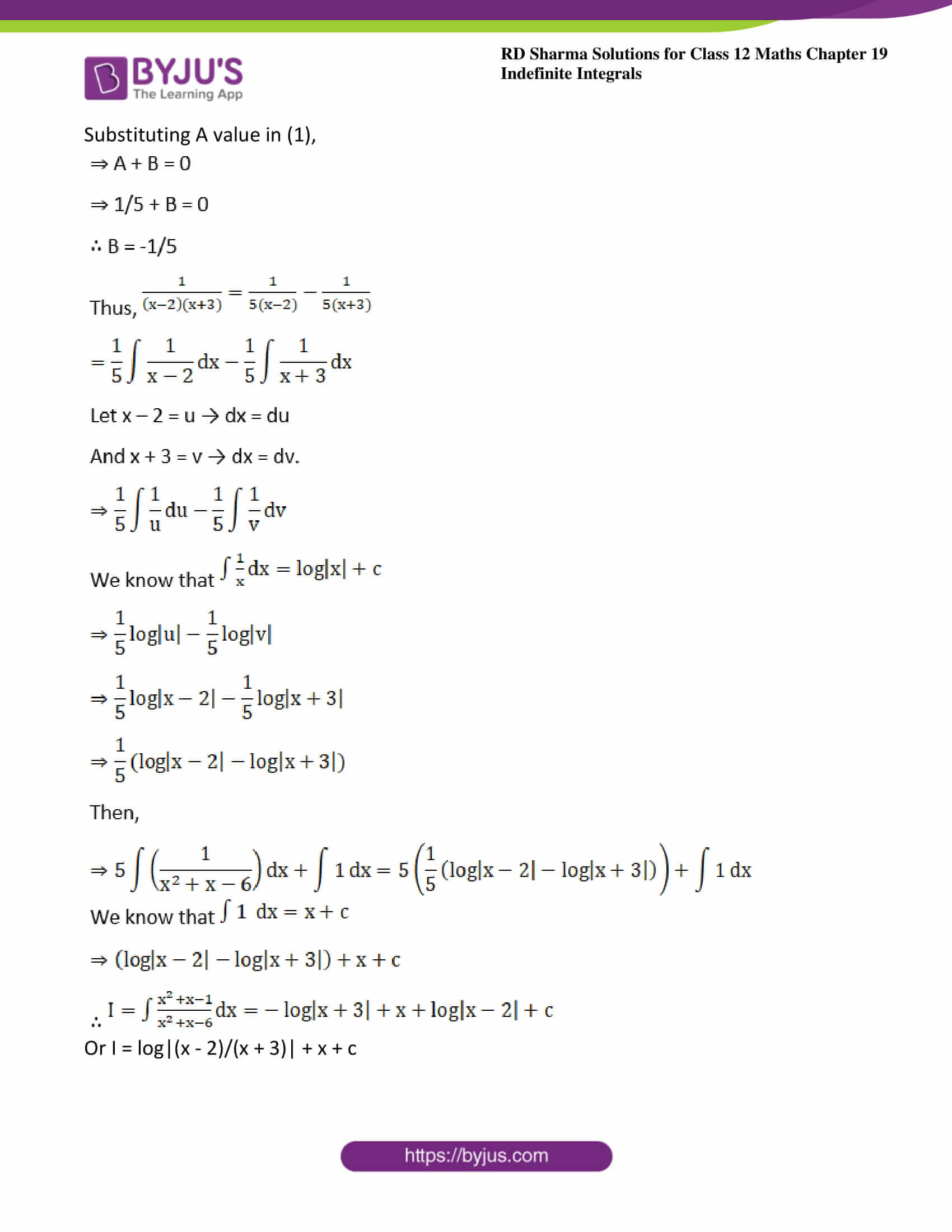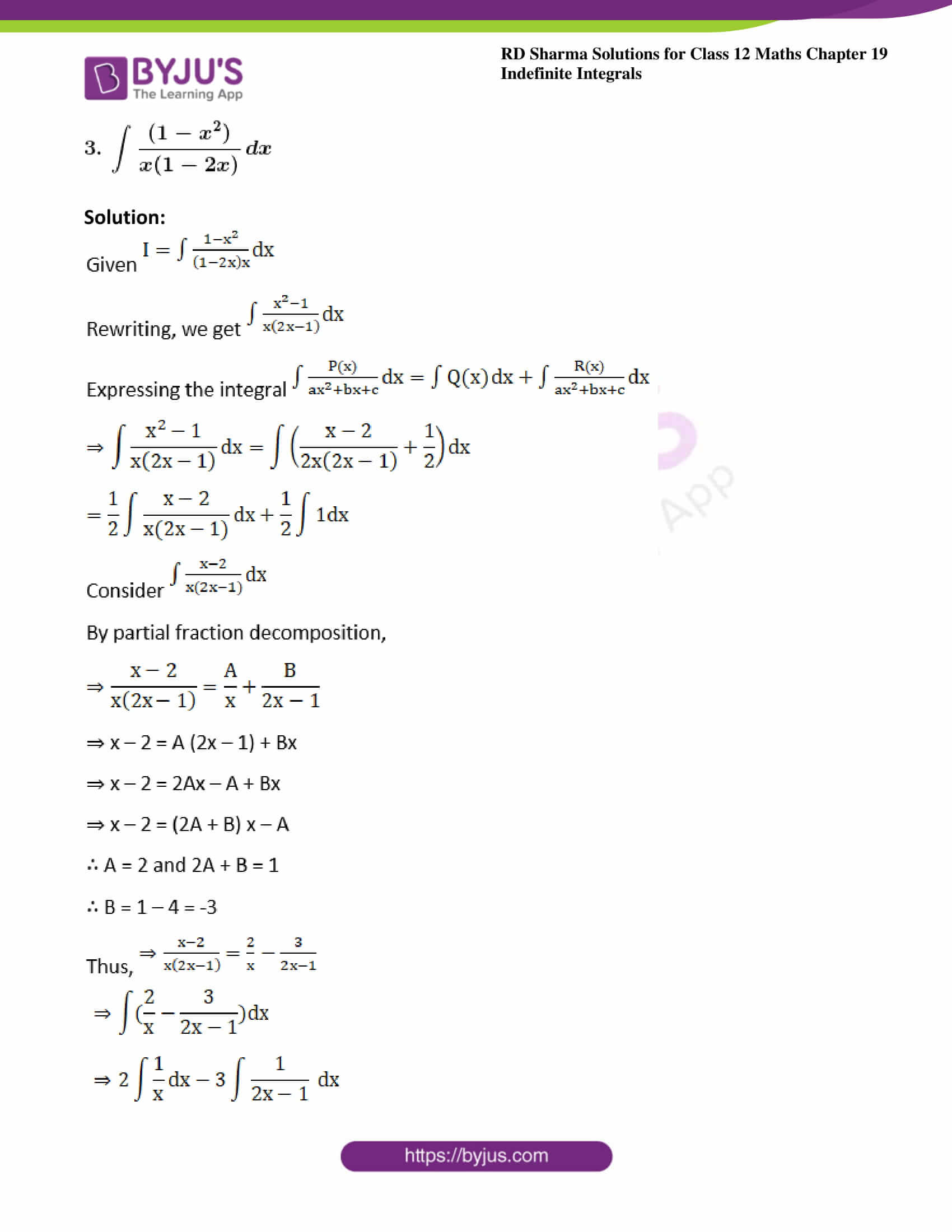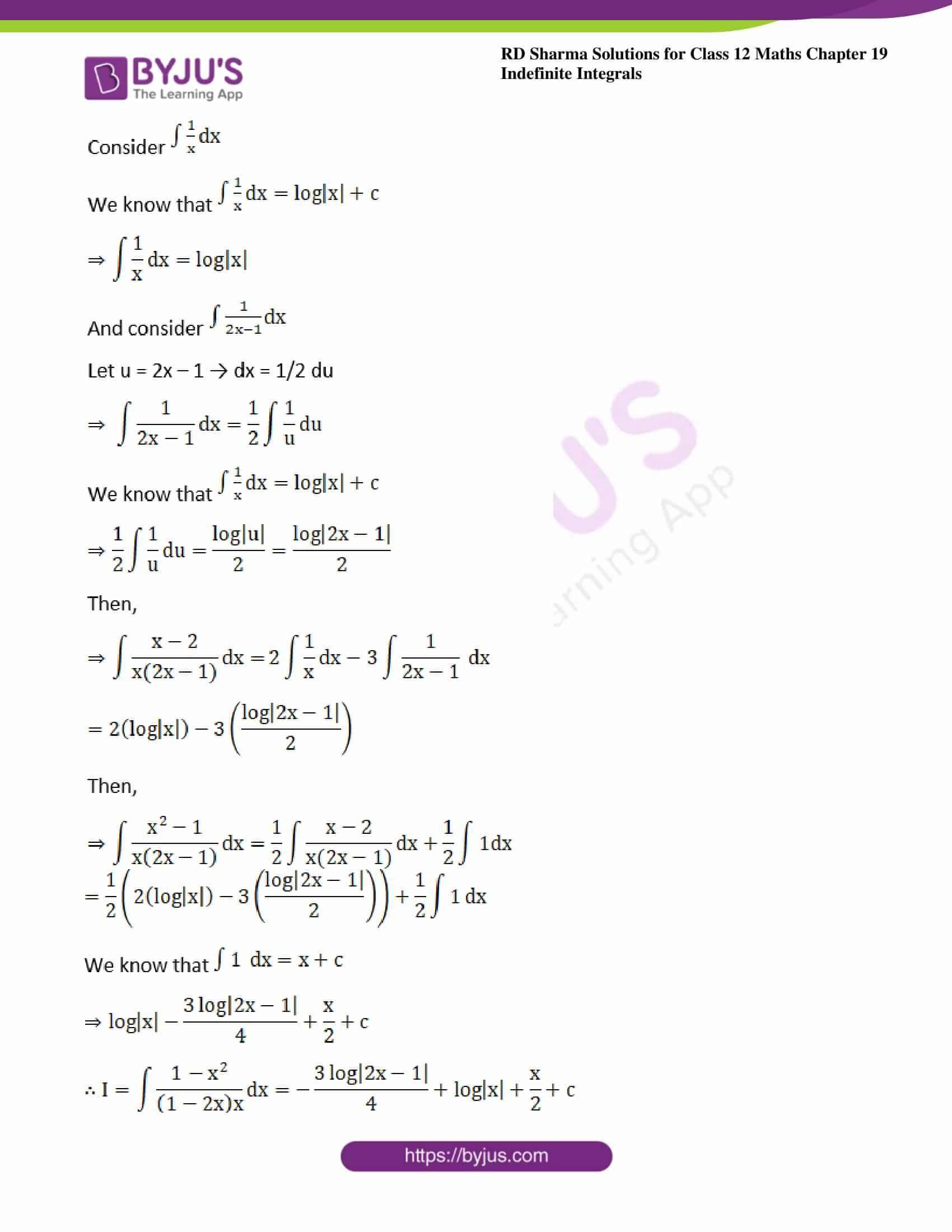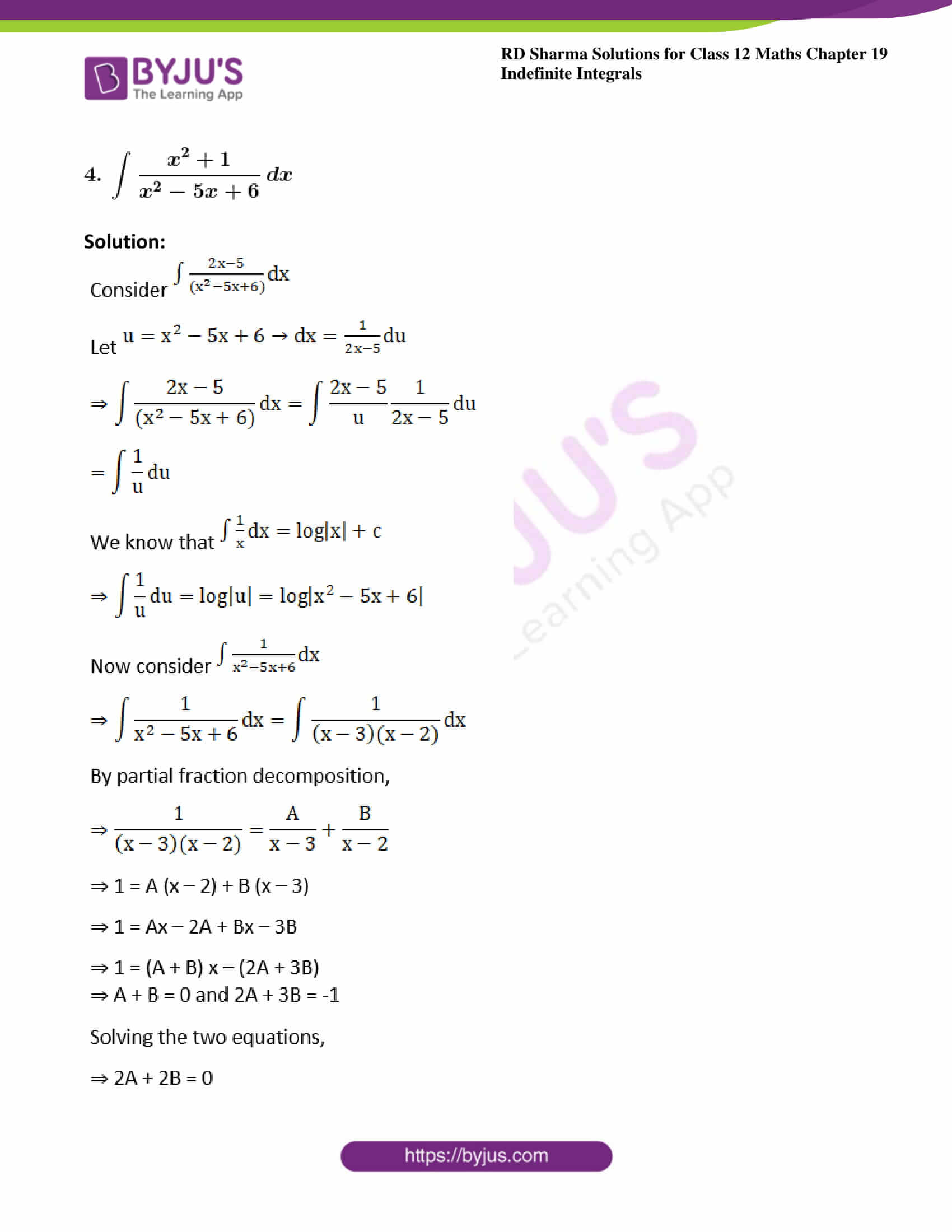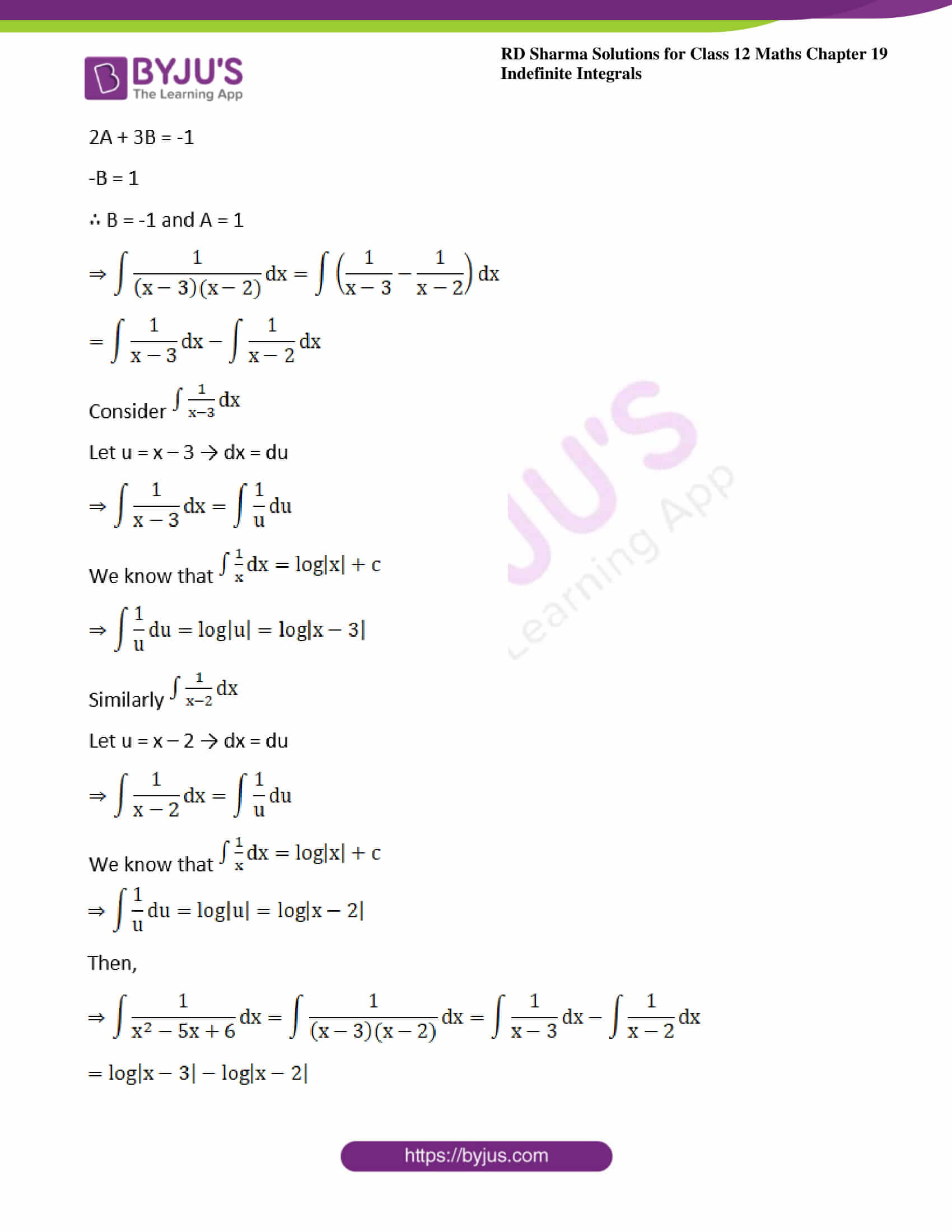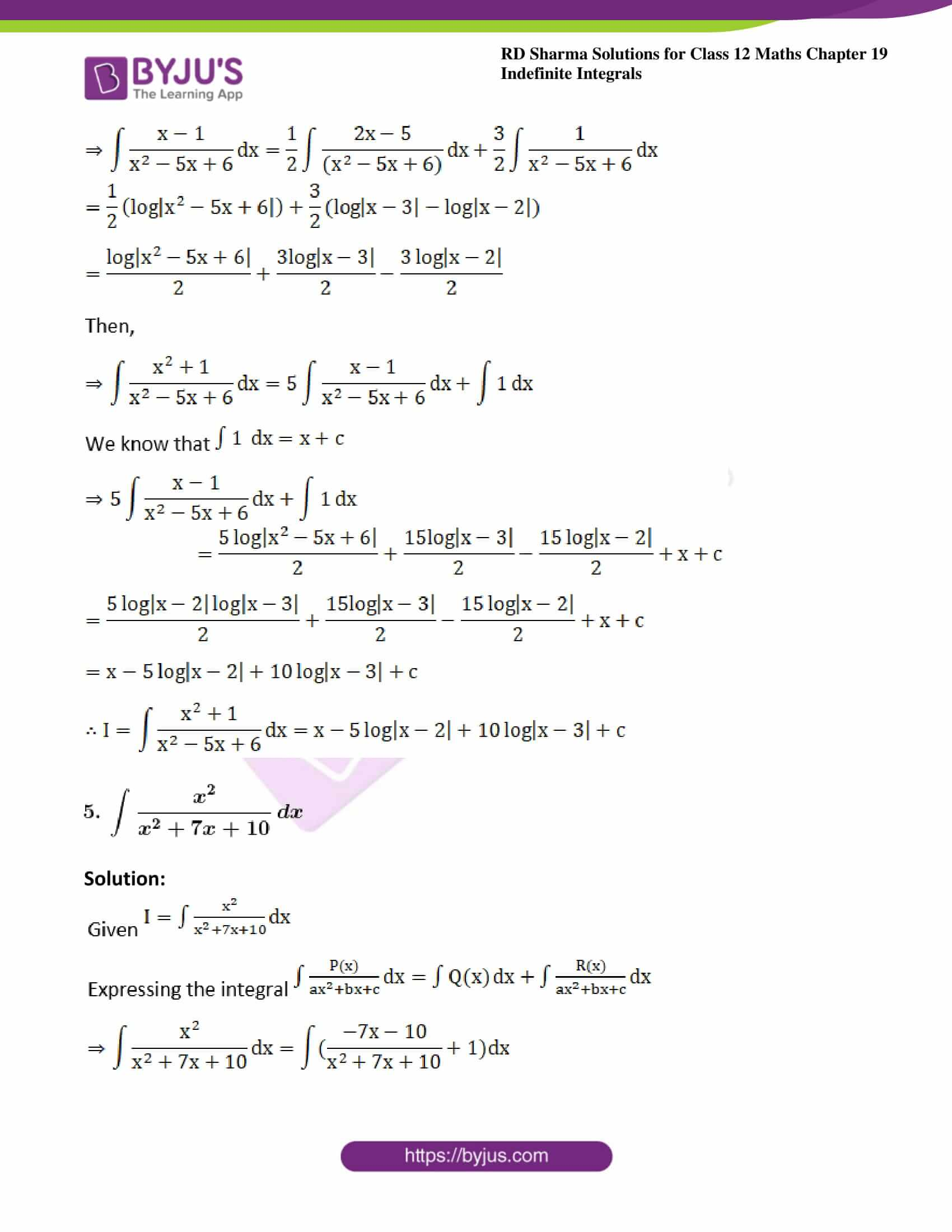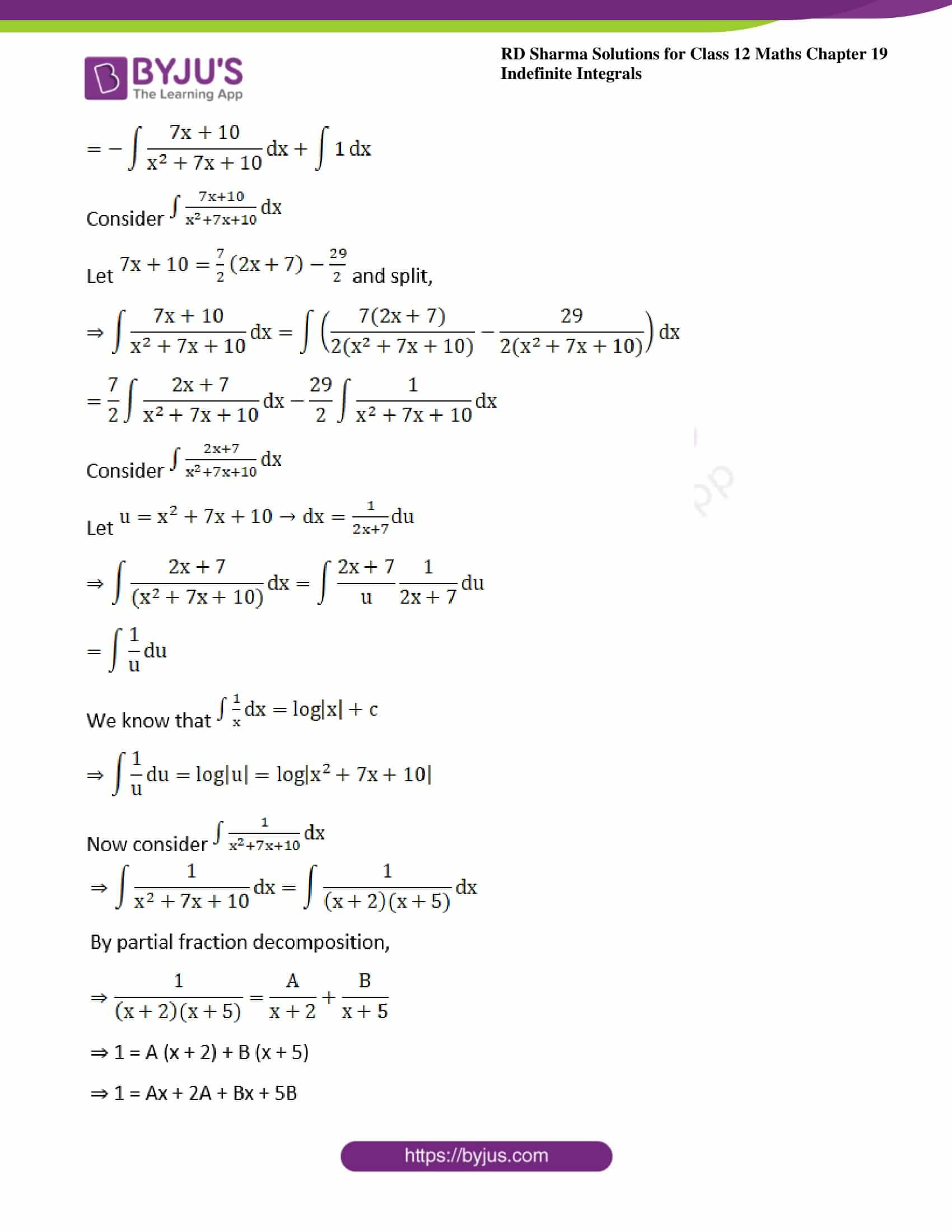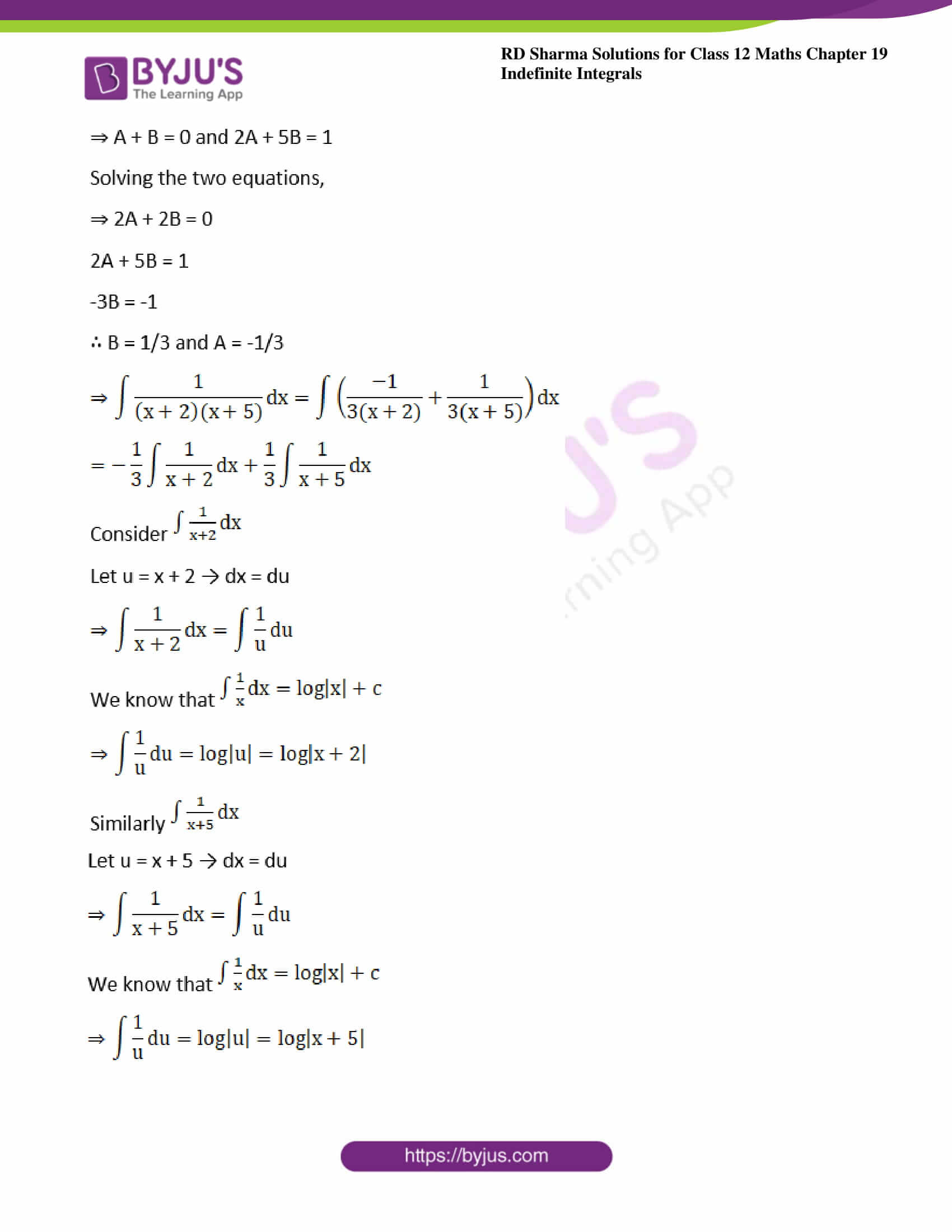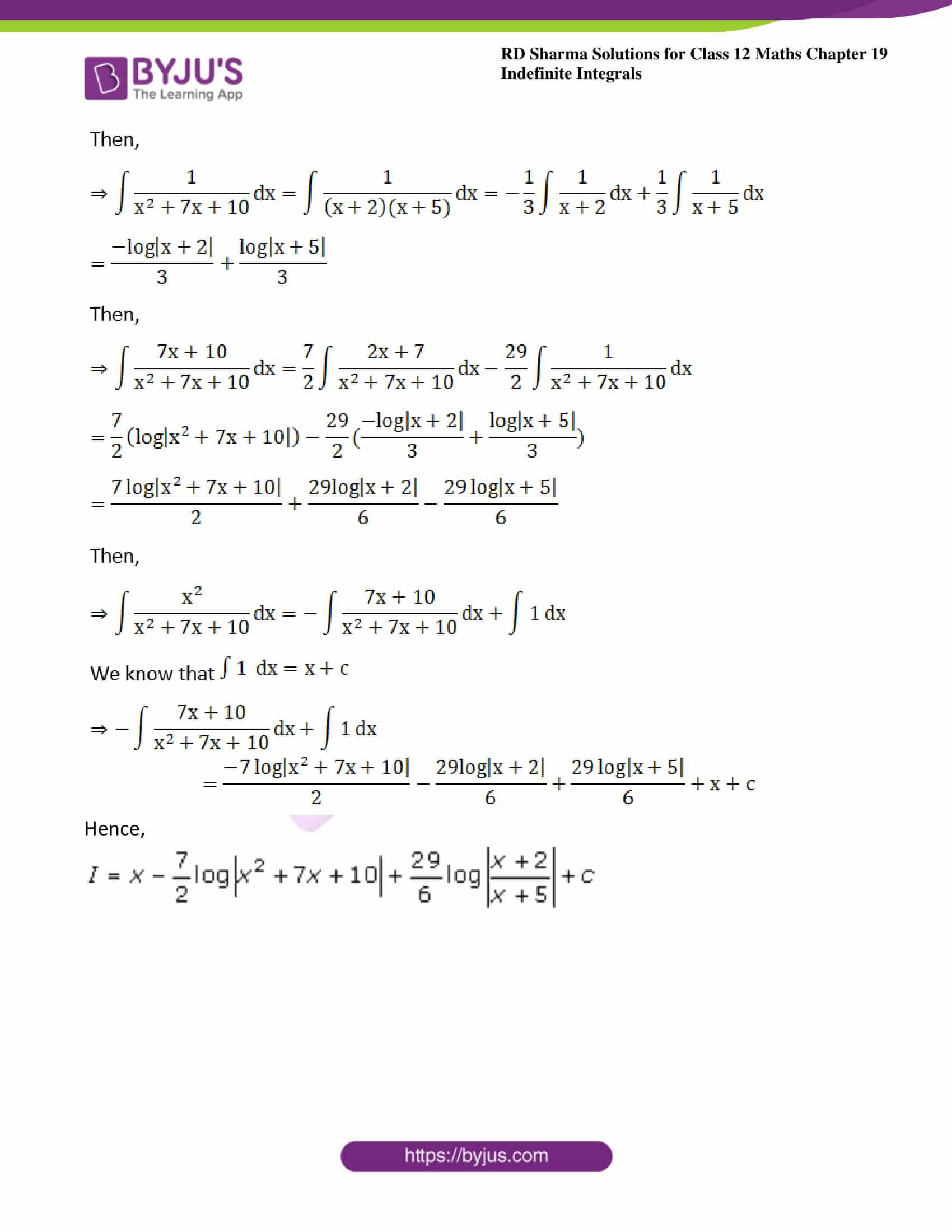### Exercise 19.20 Page No: 19.106

Evaluate the following integrals: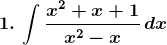Solution: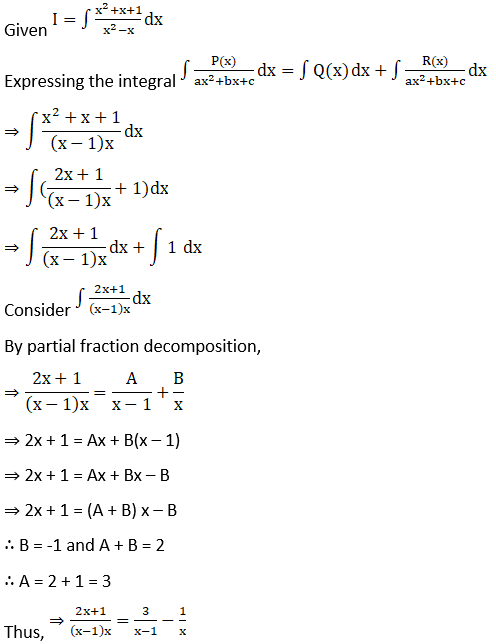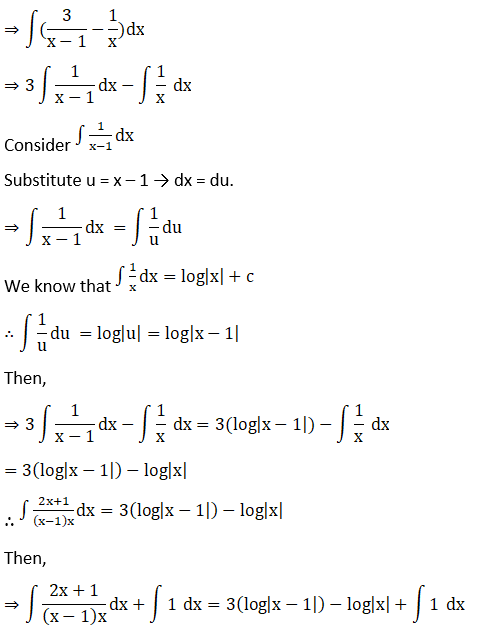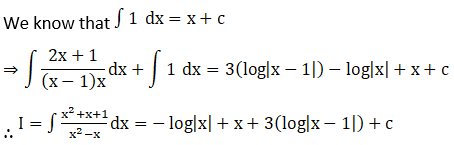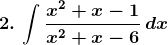Solution: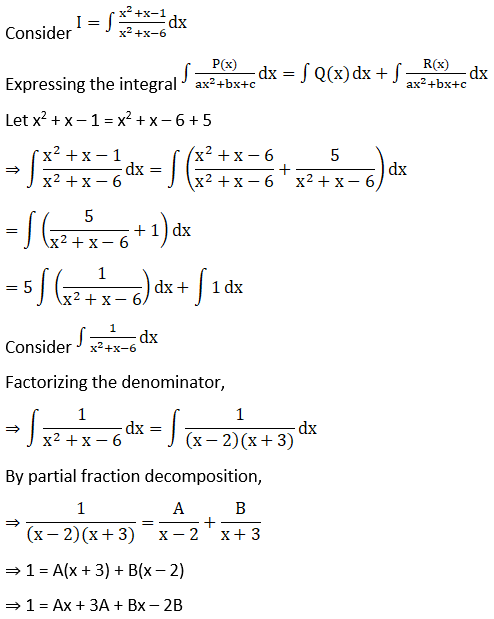⇒ 1 = (A + B) x + (3A – 2B)

⇒ Then A + B = 0 … (1)

And 3A – 2B = 1 … (2)

Solving (1) and (2),

2 × (1) → 2A + 2B = 0

1 × (2) → 3A – 2B = 1

5A = 1

∴ A = 1/5

Substituting A value in (1),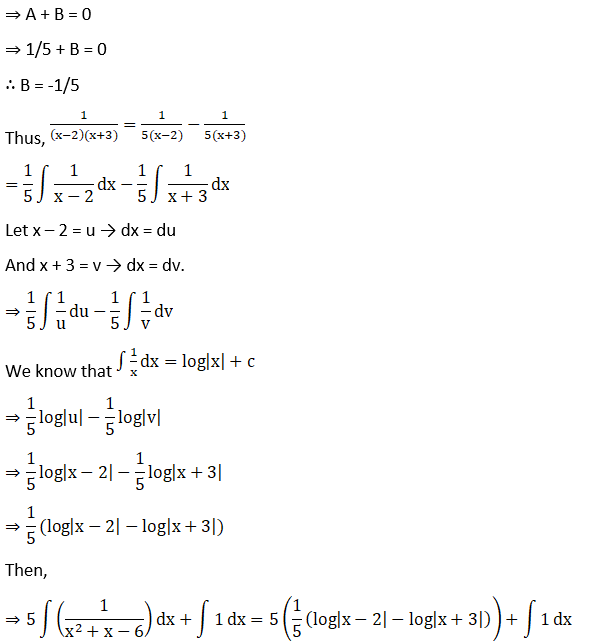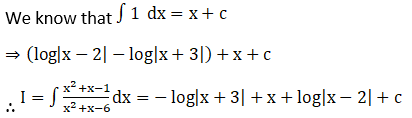Or I = log|(x – 2)/(x + 3)| + x + c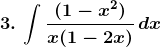Solution: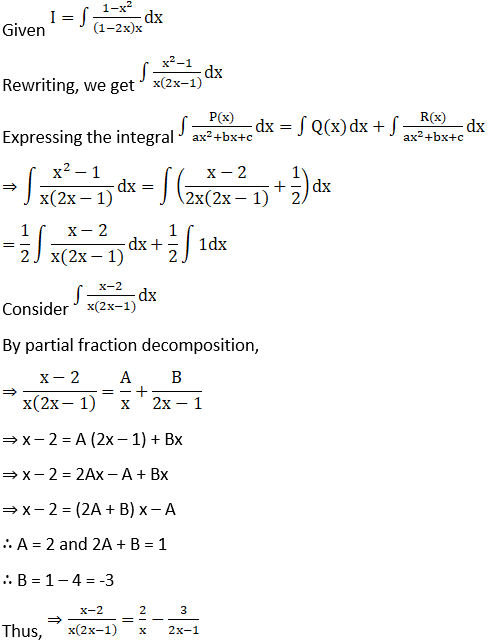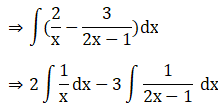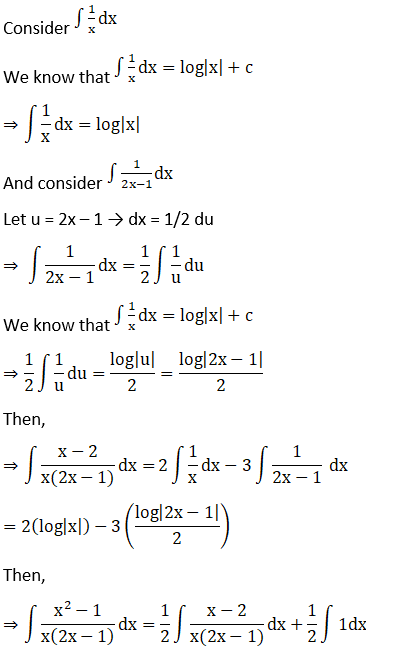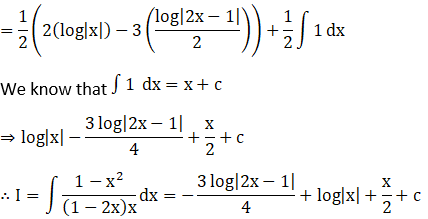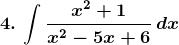Solution: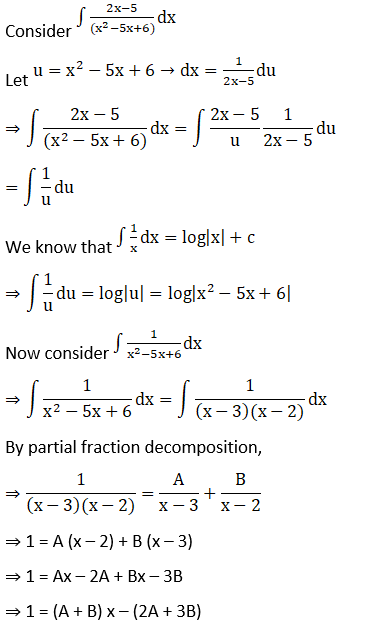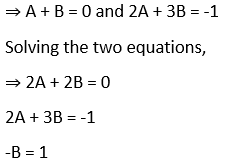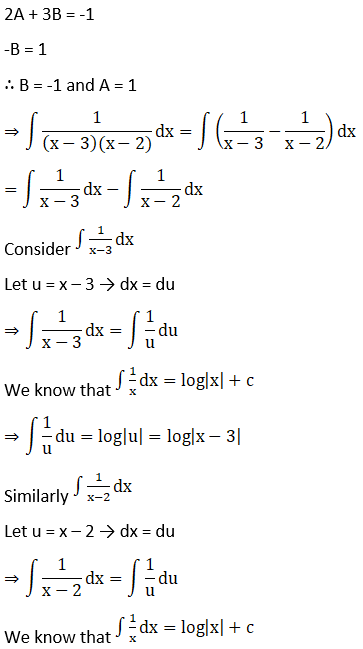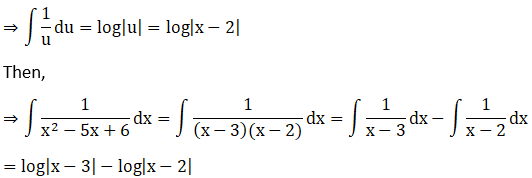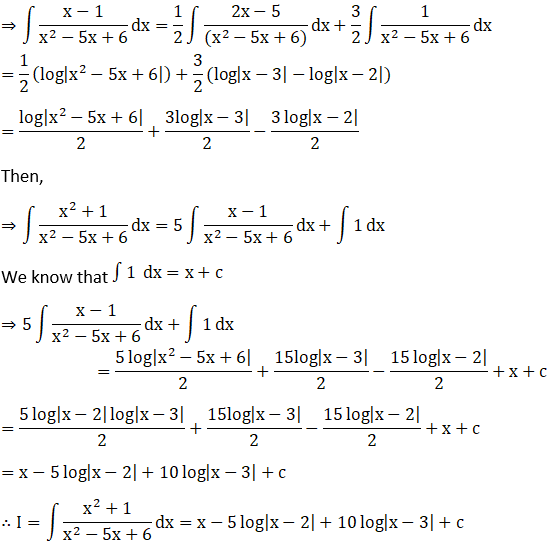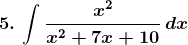Solution: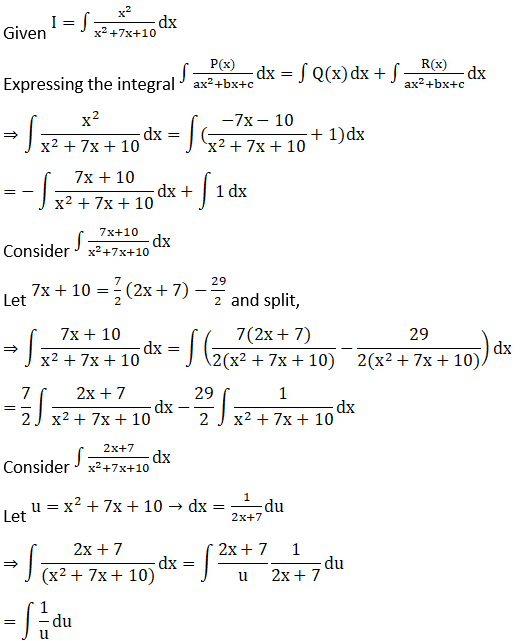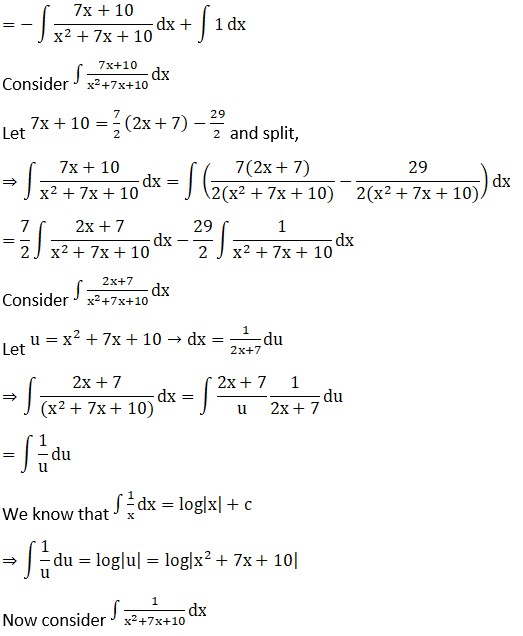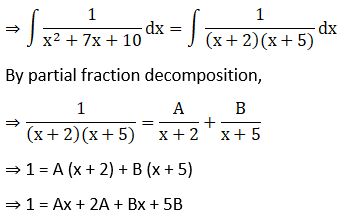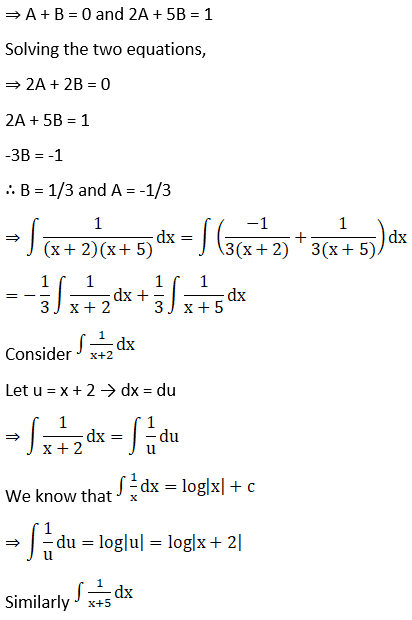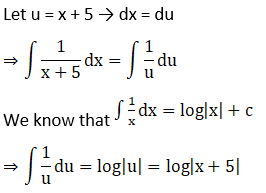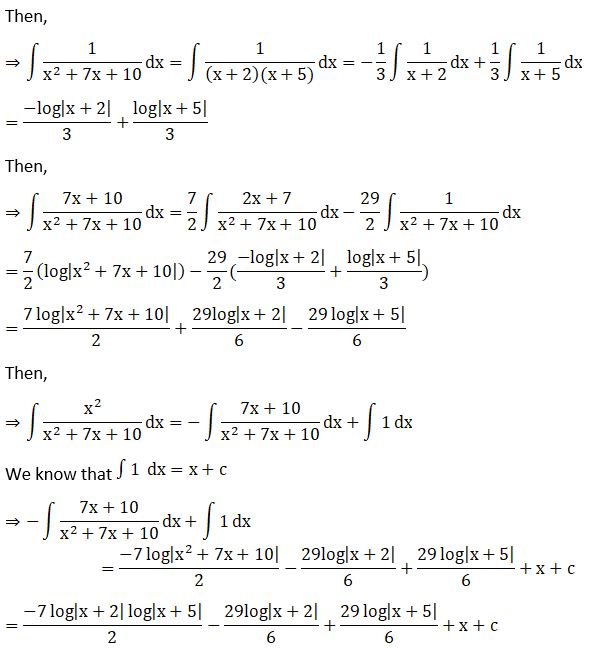Hence,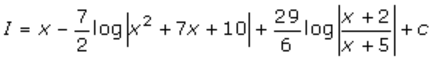### Access other exercises of RD Sharma Solutions For Class 12 Chapter 19 – Indefinite Integrals

Exercise 19.1 Solutions

Exercise 19.2 Solutions

Exercise 19.3 Solutions

Exercise 19.4 Solutions

Exercise 19.5 Solutions

Exercise 19.6 Solutions

Exercise 19.7 Solutions

Exercise 19.8 Solutions

Exercise 19.9 Solutions

Exercise 19.10 Solutions

Exercise 19.11 Solutions

Exercise 19.12 Solutions

Exercise 19.13 Solutions

Exercise 19.14 Solutions

Exercise 19.15 Solutions

Exercise 19.16 Solutions

Exercise 19.17 Solutions

Exercise 19.18 Solutions

Exercise 19.19 Solutions

Exercise 19.21 Solutions

Exercise 19.22 Solutions

Exercise 19.23 Solutions

Exercise 19.24 Solutions

Exercise 19.25 Solutions

Exercise 19.26 Solutions

Exercise 19.27 Solutions

Exercise 19.28 Solutions

Exercise 19.29 Solutions

Exercise 19.30 Solutions

Exercise 19.31 Solutions

Exercise 19.32 Solutions